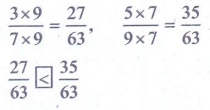Home | | Maths 5th Std | Exercise 6.4 (Comparing like fractions)

# Exercise 6.4 (Comparing like fractions)

Text Book Back Exercises Questions with Answers, Solution : 5th Maths : Term 3 Unit 6 : Fractions : Exercise 6.4 (Comparing like fractions)

Exercise 6.4

1. Write the proper symbol fromi. 3/5 > 2/5

ii. 2/8 > 1/8

iii. 2/11 < 10/11

iv. 3/1510/30

Multiples of 15 = 15, 30, 45, 60

Multiples of 30 = 30, 60, 90

Common denominator = 303×2 / 15×2 = 6/30, 10×1 / 30×1 = 10/30

6/30< 10/30

v. 3/83/7

Multiples of 8 = 8, 16, 24, 32, 40, 48, 56

Multiples of 7 = 7, 14, 21, 28, 35, 42, 49, 56

Common denominator = 56

3×7 / 8×7 = 21/56, 3×8 / 7×8 = 24/5621/56 < 24/56

Ans 3/8 < 3/7

vi. 4/74/11

Multiples of 7 = 7, 14, 21, 28, 35, 42, 49, 56…, 77

Multiples of 11= 11, 22, 33, 44, 55, 77

Common denominator = 77

4×11 / 7×11= 44/77, 4×7 / 11×7 = 28/7744/77 > 28/77

vii. 5/121/6

Multiples of 12 = 12, 24, 36

Multiples of 6 = 6, 12, 18, 24, 30

Common denominator = 12

5×1/ 12×1 = 5/12, 1×2 / 6×2 = 2/125/12 > 2/12

viii. 4/9 = 4/9

ix. 3/75/9

Multiples of 7 = 7, 14, 21, 28, 35, 42, 49, 56, 63

Multiples of 9 = 9, 18, 27, 36, 45, 54, 63

Common denominator = 63

3×9 / 7×9 = 27/63, 5×7 / 9×7 = 35/6327/63 < 35/63

x. 4/111/5

Multiples of 11=11, 22, 33, 44, 55

Multiples of 5= 5, 10, 15, 25, …..55

Common denominator = 55

4×5 / 11×5 = 20/55, 1×11 / 5×11 = 11/55

20/55 > 11/55